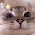# C++ Program for Shortest Job First (SJF) Scheduling Algorithm

Problem:- C Program for Shortest Job First (SJF) Scheduling Algorithm or C/C++ Program for shortest job first scheduling algorithm or Program for Shortest Job First (or SJF) scheduling or C++ Programming Codes and Concepts: Shortest Job First (SJF) or Write A Program In C++ For Shortest Job First or C program for shortest job first scheduling algorithm or C++ Program for Shortest Job First (SJF) Scheduling Algorithm.

Check This:- Hacker rank solution for Strings, Classes, STL, Inheritance in C++.

Explanation:- Shortest Job First (SJF) is a Non- primitive Scheduling Algorithm we also know SJF as Shortest Job Next (SJN). Shortest Job First (SJF) is also a pre-emptive scheduling algorithm, that means It is compulsory for CPU to know the next process how much time process will take for executing. In simple word, we can say that Shortest Job First (SJF) is executed (put on CPU priority) the only process those have a minimum time for execution.

1. What is Shortest Job First (SJF)  Scheduling Algorithm?.
2. How to Calculate Turn Around Time?.
3. How to Calculate Waiting Time?.
4. Example.

What is Shortest Job First (SJF) Scheduling Algorithm?.

Shortest Job First (SJF) is a pre-emptive Scheduling Algorithm for execution Process in Minimum time order means,  a process has a minimum time for execution execute first and then second minimum time taking process. SJF is an alternative for FCFS cause it reduces the average waiting time and also good for Batch systems.

How to Calculate Turn Around Time?.

Turn Around Time = Completion Time – Arrival Time

Total Turn Around Time = Turn Around Time / Total Number of Process

With the help of this formula, we can calculate a Turn Around Time of all process in Queue.

How to Calculate Waiting Time?.

Waiting Time = (Turn Around Time – Burst Time)

Total Waiting Time = Waiting Time / Total Number of Process.

Example:- We are taking 4 process whose CBT, or Burst Time Is 6, 8, 7, 3 respectively and the output is given below.

Enter The Total Number of Process: 4

Enter CBT of Process:
6
8
7
3

========================================================
Gantt. Chart
========================================================
P4  |  P1  |  P3  |  P2  |
--------------------------------------------------------
00    03    09    16    24

--------------------------------------------------------
Process    CBT   Waiting Time  Turn Around Time
--------------------------------------------------------
P            3           0                   3
P            6           3                   9
P            7           9                 16
P            8         16                 24

Total Waiting Time: 7

Total Turn Around Time: 13

Also Check:- Geeksforgeeks solution for School, Basic, Easy, Medium, Hard in C++.

Solution:-

#include<bits/stdc++.h>
using namespace std;

int main()
{
/*Visit - www.programmingwithbasics.com*/

cout<<"=====================================";
cout<<"\nVisit - www.programmingwithbasics.com";
cout<<"\n=====================================";
int p, i, j, sum=0, min, index;
float awt=0, atat=0;

cout<<"\nEnter The Total Number of Process: ";
cin>>p;

int proc[p];

int *cbt = new int[p];
int  *wt = new int[p];
int  *gc = new int[p];
int *tat = new int[p];
int *tmp = new int[p];

cout<<"\nEnter CBT of Process:\n";

for(i=0; i<p; i++)
{   cin>>cbt[i];
tmp[i]=cbt[i];
}

sort(cbt, cbt+p);

cout<<"\n========================================================\n";
cout<<"\t\tGantt. Chart";
cout<<"\n========================================================\n";

for(j=0; j<=p; j++)
{
min=100;
for(i=0; i<p; i++)
{
if(min>tmp[i]&&tmp[i]!=-1)

min=tmp[i];
index=i;
}
}

gc[j]=sum;
wt[j]=sum;
sum+=tmp[index];
tat[j]=sum;
tmp[index]=-1;

if(j==p)
break;
cout<<'P'<<index+1<<"  |  ";
proc[j]=index+1;

cout<<"\n--------------------------------------------------------\n";

sum=0;

for(j=0; j<=p; j++)
{
if(gc[j]<10)
cout<<0;
cout<<gc[j]<<"    ";
sum+=gc[j];
}

cout<<endl;

atat=(sum*1.0)/p;

cout<<"\n--------------------------------------------------------";
cout<<"\nProcess\t\tCBT\tWaiting Time\tTurn Around Time";
cout<<"\n--------------------------------------------------------\n";

for(i=0; i<p; i++)
{
cout<<"P["<<proc[i]<<"]\t\t"<<cbt[i]<<"\t"<<wt[i]<<"\t\t"<<tat[i]<<endl;
awt=awt+wt[i];
}
awt=(awt*1.0)/p;
cout<<"\n\nTotal Waiting Time: "<<awt;
cout<<"\n\nTotal Turn Around Time: "<<atat<<endl;
return 0;
}

Output:-

1.Anonymous3/05/2016

Thanks For Helping In This Code

2.may i ask whats all the pointers for?
int *cbt = new int[p];
int *wt = new int[p];
int *gc = new int[p];
int *tat = new int[p];
int *tmp = new int[p];